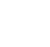# Computing the break even point

Company owners and managers constantly face decisions on selling prices, costs, and volumes.
By Bay Cabrera |

Company owners and managers constantly face decisions on selling prices, costs, and volumes. As a result, many use the cost-volume-profit analysis as an analytical tool to help them learn how costs and profits behave in response to changes in the business activity. It helps them to answer the following questions:

What level of sales must we reach to cover all expenses and break even?

• How many units should we sell to earn a given operating income?

• If we expand capacity, what will happen to our profitability?

• If we increase advertising, how much should sales increase to maintain our income from operations?

We shall focus on the use of cost-volume-profit analysis to calculate the break-even point. But first, we have to define the key terms:

• The break-even point is that point of activity or sales volume where the total revenue and total expenses are equal: there is neither profit nor loss. Enough units are sold so that the difference between the selling price and the variable costs is just enough to cover the fixed costs.

ADVERTISEMENT - CONTINUE READING BELOW

Variable costs are costs that increase and decrease directly and proportionately with the changes in volume.  If, for example, volume increases 10 percent, a variable cost will also increase by about 10 percent. Variable costs consist of the cost of goods sold in merchandising, materials and direct labor in manufacturing, as well as factory costs that are related to the rate of production such as power and water bills, repairs, and manufacturing supplies. Sales commissions, compensation, and income taxes are variable costs that are not related to production.

• Fixed costs are costs that remain unchanged
despite changes in volume. Fixed costs consist of expenses such as fixed rentals, depreciation, indirect labor, insurance, real property taxes, office salaries and supplies.

• Contribution margin is the margin of sales over variable costs.
It is the sales contribution after all variable costs have been paid to recover the fixed costs and realize profit.

Contribution rate is the contribution margin expressed as a percentage of the sales price. It represents the percentage of each peso revenue that can cover fixed costs or provide an operating profit.

ADVERTISEMENT - CONTINUE READING BELOW

METHODS OF COMPUTATION

Business owners operate to earn profits. However, there is no profit until they recover their fixed costs. The variable costs claim the first portion of the selling price of every unit sold. As a result, the residual amount of sales over variable costs or the contribution margin is the peso contribution to the recovery of fixed costs and profits, in that order.  The owner profits only when the volume is enough to boost the contribution beyond the level of the fixed costs.

The volume at which enough units have been sold to accumulate a contribution margin equal to the fixed costs, is the break-even point—the point of zero profit. If you know the unit selling price, unit variable cost, unit contribution margin, and total fixed costs, you can establish the break-even volume and determine the profits for the different levels of forecast volume. You can compute the break-even point by using the following methods:

ADVERTISEMENT - CONTINUE READING BELOW

## Latest Articles

Close